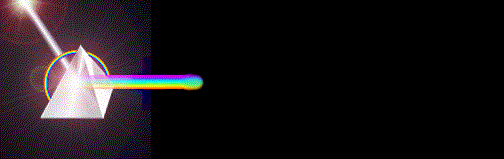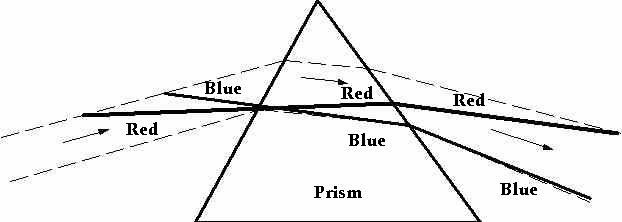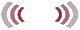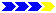Welcome to   "Science Fundamentals"   >Fundamentals of refraction<   (an article composed in the year 1990)   By: Leonard Van ZantenFundamentals of refraction

CHAPTER 49INDEX TO OTHER PAGES

1. Established fundamentals show light to refract to an index in velocity, dispersing according to the varied speed of the separate wavelengths.

2. Measurements revealing said reductions proved however to be a cloak masking a constant velocity making more time in place.

3. Hence, the dispersion of light is found not in varied velocities as was previously thought, but in waveform incidence.

Principle of refraction

1. Huygens principle of refraction is the classical example of a row of men walking obliquely into a bed of sand  The first to enter upon the bed of sand are slowed while the remainder continues until they come upon the sand, which effects the turn.

2. Correct as this in principle may be, it is wanting in the fact that it displays waves as "fronts", as lines perpendicular with the line of incidence.  And this is not the case with the wavelength of the electro-magnetic spectrum, since these in their formation are very near parallel with the line of incidence.

3. The visible spectrum is roughly from 4000 to 7000 Angstroms.  Let thus AD, figure 49-2    be the line of incidence, and let us draw the lengths of the waves to scale, increasing only the amplitude (width) for demonstration purposes.  A wavelength of 7000a would thus come according to ED. (Half-length 3500a).

4. Here we must pause, for instead of one angle of incidence, we find "two" angles of incidence by which the light strikes the water.  Consequently, the angle of refraction must be according to the sum of these two.  This in turn is by the sum of the retardation effected by the composition.

5. It is not that light is dispersed into its varied colors by any index of retardation, but by the angle at which it strikes the denser or lighter media.1. As a necessity therefore we must find a term for these two incidences to know one from the other.  Let therefore the first incidence (AD), (the line at which the whole beam of light strikes the water) be called the "linear" incidence (Li).  And the incidence of the formation of the wave, as the "waveform" incidence, or "angular" incidence (Ai).

2. The comparative wave angles for the red at 7000a, and for the blue at 4000a, are ED and GD respectively.  The index of water then being 1.33, the red wave will be compressed to have a new crest to crest measure of 5250a, while the length for blue light is reduced to 2970a.

3. This will give each of them (as they are submerged in the water) a "new incidence" noted DL for the red and DK for the blue.

4. If these same new angles passed upon the water from the "normal" (straight down) they would merely continue to pass straight on through with nothing more than a compression placed upon them, their waveform incidence changing from ED to FD, and from GD to HD.

5. The important factor to be noted here is - that the first incidence is not alone, or single in determining the actual angle of refraction since it is paired with (what we might call) the real incidence, namely the waveform incidence.

6. This change in direction from the one media to the other, to our eyes, is a definite change, and also to the first line of incidence, yet it is a zero change in the wave itself noted HDK (blue wave), and FDL (red wave).

7. The dispersion of the light thus is not in a change of velocity, nor in any varied velocity whereby these wavelengths might travel through the denser medium, but in incidence, specifically, "waveform" incidence.

8. The composition is no different for light whether it comes in a 4-cm length or in a 7-cm length, or any length.  Nor shall it transpose any wavelength at any other speed than any other wavelength since the velocity is endowed upon them by the engines of the media at a single constant, which may be called "the speed of light c" or as I call it, "the constant".

9. The dispersion of light then is directly proportional to the sum difference in the wavelengths that are passed in some incidence other than the normal upon a surface in which it may refract.

10. If for example, we scaled the wavelengths from 4000a to 7000a on a piece of paper, and the sum separation came to 15 degrees, a beam of light containing wavelengths in these perimeters will always disperse by that 15 degrees. These 15 degrees then are at all times equal to the 1500 angstroms difference in the wavelengths from 4000 to 7000.

11. When for example we change the first incidence, it will have no effect upon the degree of dispersion; it will remain at 15 degrees.  And however much blue or red shift (compression or expansion) we may place upon the light, it will not affect this sum difference of the dispersion that we measure.

12. All this is evident from the form of the wave in the angle of incidence at which it is brought to bear.  When a sum difference of 10 degrees is brought to bear, the resultant dispersion will not be any greater nor smaller than these 10 degrees.

Incidence paired with velocity

1. I am going to include velocity in the index of retardation as joined with incidence in determining the angle of refraction.

2. But in all such reference this will merely be a "figure of speech," since velocity as such - has no relevance to how or why a wave is refracted, or varied in speed from any one composition to another.

3. It is the composition, which effects apparent velocity, that I prefer to call "Relative" velocity.  And the single factor of the composition which effects these changes is "density", which we could also spell as; the effective distance between atoms.

4. But now you will say; that all this is common knowledge, that we knew all along that retardation is effected by the composition in the density thereof, and that velocity is effected on account thereof.  ...  But I am prepared to show that your judgment is somewhat in error.

5. If I quote what is stated under "refraction" in the textbooks of science. "The path of a wave bends "because" the wave travels with different speeds in the two media's."

6. This clearly shows that we place the cause on velocity, which is incorrect.  But if by velocity as a figure of speech we understand a change in wavelength (shift) due to a change in the relative distance of atoms from one to the other (density), we may accept it as such

7. Illustration figure 49-3 is the correct procedure by which to show the principle of refraction.  It shows both incidence's, the first, as also the waveform, as well as the compression of the wave by velocity as it enters from one density into another.

8. The linear incidence is tangent AB.  The angular incidence are, AK and AX, both of which can be varied simultaneously by tangent AB, but not by velocity, unless they already came by a change in their tangents due to velocity.Click on illustration for larger view

1. An example of this is when instead of coming from air upon a glass prism, the light entered upon the prism from a composition such as water.  In other words, the light already passed through compression.  Which of course can also be said for the light coming from air as it passed through compression coming from space.

2. The compression factor then is shown as "velocity" parallel with the line of first incidence.  Tangents KL and XN then reflect the sum retardation, the change in wavelength, which in a straight line show both the angle of refraction and the degree of dispersion.

3. Wherefore in conclusion, it is the line with "least angular moment" that is least refracted, the one most parallel with the line of incidence.

4. Whenever light is refracted as per illustration above, it turns towards the normal entering a denser media and away from the normal entering a lesser dense media.  Moreover it seems to me that light turns by a clockwise rotation, not that I am positive, but it seems that way.Defining a wave into velocity

1. But there is much more to discover in the nature of waves to learn why blue or red waves should be longer or shorter.  Or why there should be a difference in the velocity at which they may proceed through our media, if any.

2. Or why there is no real change in velocity, and that such change is a sight deception, or as I call it, a "relative velocity" which is not really the speed of light, but only a measure of distance in time.

3. The four properties of light then are length, frequency, velocity, amplitude, intensity, and its direction of propagation.  All four are directly proportional to one another.

4. And while I said four, but named six, this is because intensity is not really related to amplitude, but synonymous with frequency.  The direction of propagation also is not really a property of light but of another fundamental factor in nature.

5. Let us thus put aside our two dimensional concepts of waves, and duly consider that nature is three dimensional, and that it cannot be thought of in any other way.  Striking a single line will place length width and depth; neither single nor any two dimensions are found exclusive.

6. In finding the velocity of light therefore, it must be in these fundamentals, "length", "amplitude", and "frequency".  Length and frequency are well known, but how may the amplitude of a wave be found?

7. If we have two factors we can always find the third, but with one factor alone it becomes difficult yes, but not impossible.

8. A riddle posed to Marilyn Vos Savant read; "Mary is 24 years old.  Mary is twice as old as Anna was when Mary was as old as Anna is now.  How old is Anna?"

9. The answer here is, to forget that Mary is 24, and first find an "arbitrary" number by which the computation might succeed.  Presuming a figure of 16, which would place the age difference at 8, and that subtracted from 24 is again 16.

10. This same reverse process works in finding the amplitude of a wave.  Taking the speed of red light at 299.905 km/see in air, and utilizing an arbitrary measure of 2.2a for amplitude, we add wavelength to amplitude divided by (c).  (c, now is the notation for the speed of light in free space, with a value somewhat below 300.000 km/sec.

11. In my reference however (c) will always hold the value of 300.000 km/see, in free space or anywhere else, the reason for which I shall explain later).

12. The division then is by 300.000, which produces the number of waves in one second of time, and multiplied by the length of the wave results into the "relative" velocity of light.  For example, and to simplify it by substituting meters for angstroms.   (L=length.  D=amplitude.  F=frequency.

L 7000 + D 2.2: c = 42843 X L 7000 = 299.905.

L 4000 + D 2.2: c = 74958 X L 4000 = 299.835.

(Index of water 1.33)

F 42.843 X L 5250 = 224.928 km/sec (red light)

F 74.958 X L 3000 = 224.874 km/sec (blue light)

F 74.958 X L 2970 = 222.625 km/sec (blue index 1.34.

1. Since it is conclusive that there is no force or composition in nature that can act as a resistance to the movement of light in its wave formation, there must for all reality be a "constant" velocity of light.

2. If a light-wave is not either captured by its reverse harmonic in the element that produced it, or brought to a halt, it must pass through any density at full speed.

3. Plainly speaking, whether or not the wheels of a conveyer belt are spaced 5 inches or 10 inches apart does not impair the velocity of the ten feet long tube being sped forth by their momentum.

4. Or to put it in other words, Einstein's equation E=Mc2 is nothing more than stupidity, Vc, or V3, or simply c, stands for the constant of velocity at 300.000 km/sec. Nor does the velocity in any atomic explosion exceeds V3, nor can matter be converted to motion, all such is but the ignorance of the blind.

Relative velocity of light

1. But with all this we also have those velocities of light which we painstakingly measure as distance in time, the "relative" velocity which are less than c.  Before all else then let us realize that waves are not transverse but circular moving by a three dimensional form.

2. And that the pathways of these waves shall only be found along the perimeters of the atoms and molecules along which they pass, since it is at these perimeters that they find their momentum from the wheels of nature.

3. By illustration figure 49-4, "M" represents the momentum.  From point A to point C the light will travel at a given velocity which we must measure or calculate from the three components length, amplitude, and frequency.

4. The timing for that distance shall be one second, so we may discover at what speed the light really traveled as it went by two directions simultaneously, linear AC, and angular AD, passing from A over B to C, in the three dimensional form of a coiled spring.

5. The shortest, and also the fastest track now is a straight line, but since light comes in the form of "waves" it cannot be found in any measure to travel the full velocity of its constant (300.000 km/see).  The little quanta (q) or the whole train of little quanta's however do travel at that speed.

6. The reduction in velocity then as a measure between AC is equal to the amplitude of the wave into the other two components of its mathematics.  That velocity then is not a real velocity of light, but a - measure of distance in time.

7. If then the amplitude is changed by a compression into a composition where the wheels are spaced closer together, the waveform being forced to increase the angular component for its means of propagation either one of two things must occur.

8. It must be stretched to maintain its 7000a length, or assume a lesser crest to crest measure, as illustrated by figure 49-5, EFG. (the latter being the case)

9. Since then the very length of a wave is it "identity," (presumed) which remains unchanged under all conditions, the new crest to crest measure is just one factor of essentially two factors that make for the "actual" length of the wave, namely, amplitude along with the crest to crest measure.

10. Consequently, when we measure the timing in which this wave of light transposes a distance of 7000 Angstroms (its former 7000a measure reduced to 5250a), it will show a greater period of time, or as we are won't to conclude, a slower velocity.

11. It shall therefore be an erroneous conclusion to state or assume that the light itself traveled slower, since in fact it did nothing, more than (as we say) make more time in place.  Distances ABC, and EFG, (Figures 49-449-5), are equal.

12. Assuming then that 5250a is correct for measure KG, the 7000a measure of AC should of course be somewhat under 7000a, (ABC being the actual 7000a measure).

Conclusion

1. While light is measured to travel from one point to another, that velocity therefore shall be "RELATIVE".  The fundamental velocity then is always greater, and found at a "CONSTANT" by which all electro-magnetic waves are propagated.

2. The refraction of light, and the consequent dispersion must be credited to "INCIDENCE" first and foremost, and to the sum retardation, which is found in the "CHANGE" of "incidence " due to compression and/or expansion of the wave, also called, blue or red shift.

3. As then figure 49:5 EFG is the case, it implies that light waves as such might not be continues, but discreet entities on the move, and to bring more and more entities on a line we arrive with lasers.

4. If within 1 km there can be more than 142 million lengths of 7000 angstrom waves, and yet only 2 of them are present, the number of lengths that pass into our eyes will be 600.000 in each and every second of time, since the speed of light at 300.000 km/sec is bringing two wave lengths for every single kilometer thereof.

5. If then for the 3600 times off and on revolutions of our standard light bulbs we assume it as being continues, how will even a single wavelet of light in every one thousand miles be anything but continues to us in our beholding?

6. Light waves as such therefore need never be continues, and if it were - how bright would it be to look into, seeing how even in the line of our strong lasers they may very well be 60 percent open with only 40 percent filled?

Here is something to think about.

ABSOLUTE PERFECTION

1. We know that the amplitude, or circular diameter of all light-waves are right around the diameter of the lighter elements which according to scientists is about one angstrom.

2. Then considering that there are ten billion angstroms in a meter, or ten million in a millimeter, there can easily be a million atoms in any one millimeter counting in the spacing that exists between them.

3. This holds in that there is room for 10 million light-waves side by side, yet with only a one million atoms there is never more than one million light-waves able to pass upon that single millimeter.

4. If then we consider that single millimeter as no more than a pencil line, and how faithful these light-waves, in their diameter of no more than a ten-million, by their minute angular moment refract so perfectly.

5. How utterly small is a one angstrom slanted line with no more than a 15 degree difference from what projects to us being either red or blue.  These after all when encountering any surface out of a direction into the normal redirect their paths most faithfully.

6. What therefore is so astounding to me is, not only the minute size of them, but how even more minute the angular differences are between what we behold as red or blue. And with that I am astounded at the complexity of our spirit as well to recognize all such minute differences.

7. How inconceivably wise and capable our Creator is, to have made all these in such minute dimensions, and from it by multiples formed the whole of the universe.  Nor did He do so with tools, as we are wont to do, but simply by the power of His word alone.

8. To whom therefore shall we liken Him?  There is no likeness of Him, nor are we able to conceive Him in any way at all for greatness and for glory, as well as for His magnificence.Next page